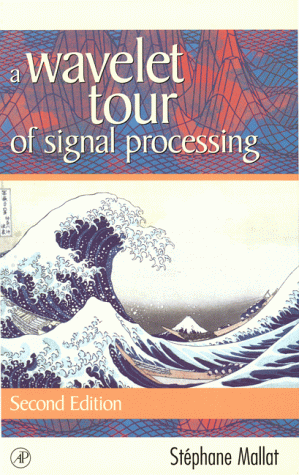# Stéphane Mallat• Wavelets have opened the door to a flow of new ideas and algorithms that have invaded most traditional fortresses of signal processing. This book travels along the bridges between applications, algorithms and theorems, with an emphasis on intuitive explanations. It is organized as a textbook for electrical engineering and applied mathematical classes, with exercices of various levels.
• All algorithms and figures are implemented in wavelet signal processing toolboxes that are freely available on the Internet, to let readers and students make their own numerical experiments. WaveLab is a Matlab toolbox elaborated at Standord University. Lastwave is a toolbox with subroutines written in C, with a friendly shell and display environment, elaborated at Ecole Polytechnique.

# New to the Second Edition

• Optical flow calculation and video compression algorithms
• Image models with bounded variation functions
• Bayes and Minimax theories for signal estimation
• 200 pages rewritten and most illustrations redrawn
• More problems and topics for a graduate course in wavelet signal processing, in engineering and applied mathematics

# Specify in the Order :

• A Wavelet Tour of Signal Processing, by Stéphane Mallat
• ISBN : 0-12-466606-X